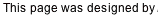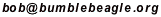## Solution Guide (making use of the strings)

In this discussion, it will be useful to refer to the bulbs with single letters (see the list below). It will also be useful to refer to a cell in the square with a lowercase letter for a column, and a number for a row. Regions will be refered to by the letter of the hint bulb given in that region. Hint blubs are circled in these diagrams so you can keep 'em straight. We'll number the strings from top to bottom, 1 thru 7.

Text will refer to the diagram above it.

A stands for an aqua bulb (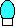).
B stands for a blue bulb (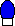).
G stands for a green bulb (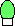).
O stands for an orange bulb (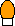).
P stands for a purple bulb (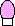).
R stands for a red bulb (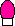).
Y stands for a yellow bulb (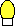).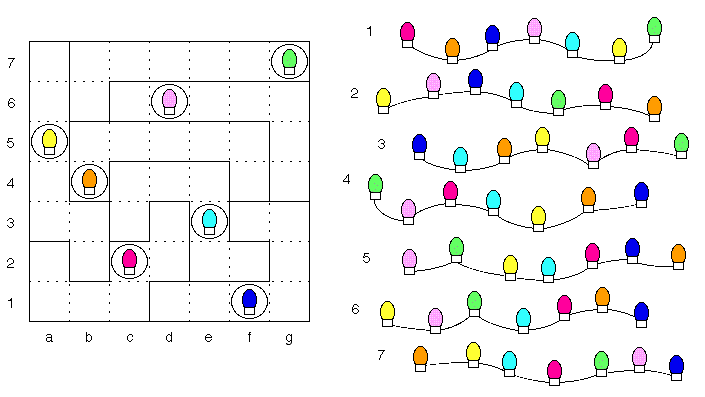So here's what we start with. As an example of the terminology, cell e3 contains an A, and cell d3 is part of region R.

Region Y needs a string with a Y two from the end. Strings 4 and 5 are the only strings that will fit. But using string 5 would put an O in b2, and column b already has an O. So string 4 belongs in region Y.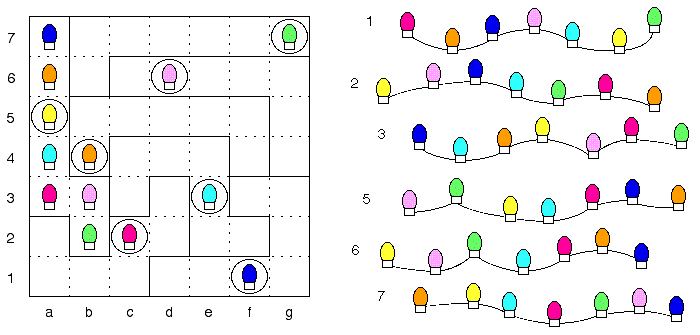Region G has a G at one end, and strings 1 and 3 are the only ones left that can provide that. But string 1 won't fit at all. Its A would go in e7, which conflicts with e3; its P in d7 conflicts with d6; its B in c7 with a7; and its O in b7 with b4. So string 3 belongs in region G.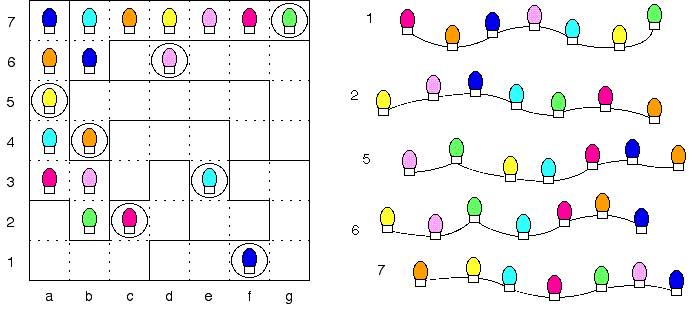Region R needs a string with an O at one end, and there are three left (strings 2, 5, and 7). Column 2 needs a Y and an R, and since row 5 already has a Y, b5 must be R. String 2 is the only one with an O-R on the end, so it belongs it region R.String 1 is the only one left that can fit region B. Either string 6 or 7 might fit region P, but string 7 would put B in c6, while row 6 already has a B. So string 6 belongs in region P.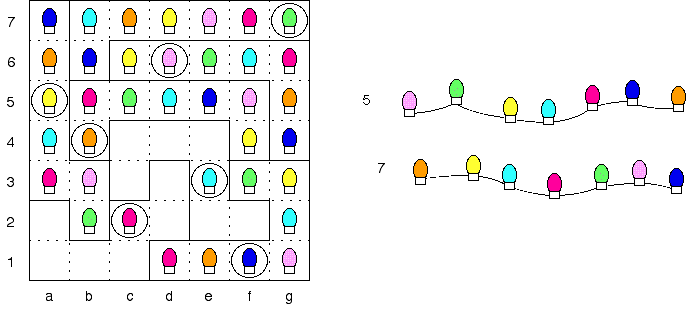The remaining two strings can only fit the two empty regions in one way; string 5 goes in region R and string 7 goes in region A.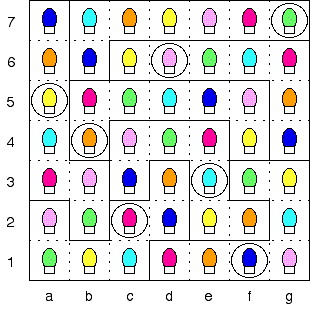Puzzle solved!

Back to Rooftop Lights Puzzle